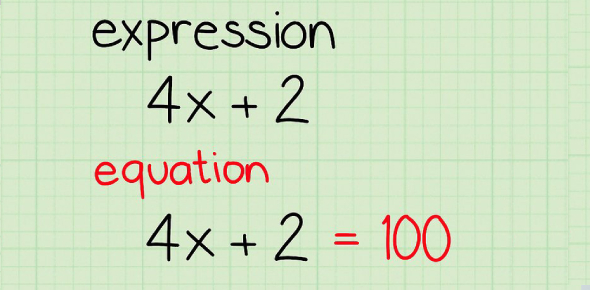# Translating Algebraic Expressions

13 Questions | Total Attempts: 350SettingsTests students ability to write an algebraic expression with in place of the word expression.

Related Topics
• 1.
2 times 7
• A.

2 * 7

• B.

2 + 7

• C.

2 / 7

• D.

2- 7

• 2.
Which of the following represent -?  Check all that apply.
• A.

• B.

Minus

• C.

Divide

• D.

Without

• E.

Product

• F.

Ratio

• 3.
The sum of 6 and a number
• A.

6 + 8

• B.

6 - n

• C.

6 * n

• D.

6 + n

• 4.
How do you write 5 * z in algebra?
• A.

5 x z

• B.

5/z

• C.

5 ^ z

• D.

5z

• 5.
Fill in the blank: The difference of 16 and a number.  (Use "n" as your variale)
• 6.
200 times a number divided by 2
• A.

200 * n/2

• B.

200 * n/3

• C.

200* 3/n

• D.

200/3 * n

• 7.
fraction
• A.

+

• B.

-

• C.

*

• D.

/

• 8.
Copy
• A.

+

• B.

-

• C.

*

• D.

/

• 9.
Increased by
• A.

+

• B.

-

• C.

*

• D.

/

• 10.
Quotient
• A.

+

• B.

-

• C.

*

• D.

/

• 11.
Sum
• A.

+

• B.

-

• C.

*

• D.

/

• 12.
Plus
• A.

+

• B.

-

• C.

*

• D.

/

• 13.
The product of
• A.

+

• B.

-

• C.

*

• D.

/